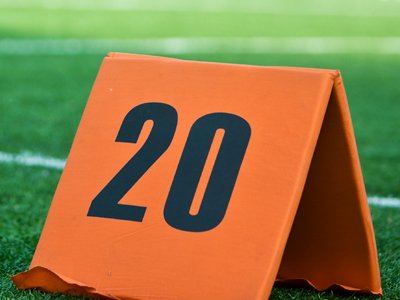2 × 10 = 20.

# 2 Times Table

You may think that the two times table is too easy but it can't be stressed enough how important learning it is. You may think you will never need to use it in life - but think again! It's essential that you know it for your 11-Plus exam.

The two times' table is important as multiplying by 2 is the same as doubling a number. The time taken for something to double, the doubling time, is an important piece of information in a lot of practical situations, e.g. working out inflation, population growth and compound interest.

Don't be too confident. Yes, the two times table is probably the easiest, but if you take it for granted then you may misread a question and get the answer wrong. Take your time and read each question carefully. You wouldn't want to fail such an easy test now would you?

So, if you really have mastered your two times table, see if you can double your scores in this quiz.

1.
2 × 7 = ?
10
12
14
16
There are 14 days in two weeks - a fortnight
2.
2 × 6 = ?
16
14
10
12
Another name for 12 is a dozen
3.
2 × 3 = ?
8
4
6
5
6 is also known as half a dozen
4.
2 × 9 = ?
18
19
14
16
One way to work this out is to multiply 2 by 10, which is 20, and then subtract 2. This gives the answer 18
5.
2 × 5 = ?
12
8
12
10
To multiply a number by 5, first times it by 10 and then halve the answer
6.
2 × 12 = ?
22
24
26
20
There are 24 hours in a day
7.
2 × 4 = ?
6
8
4
12
Remember - to multiply a number by 2 just double it
8.
2 × 8 = ?
16
12
18
14
16 is also 4 squared
9.
2 × 2 = ?
1
6
8
4
When we multiply a number by itself we square it. 2 squared is 4
10.
2 × 11 = ?
14
18
22
26
In bingo, 22 is called 'two little ducks'
Author:  Frank Evans